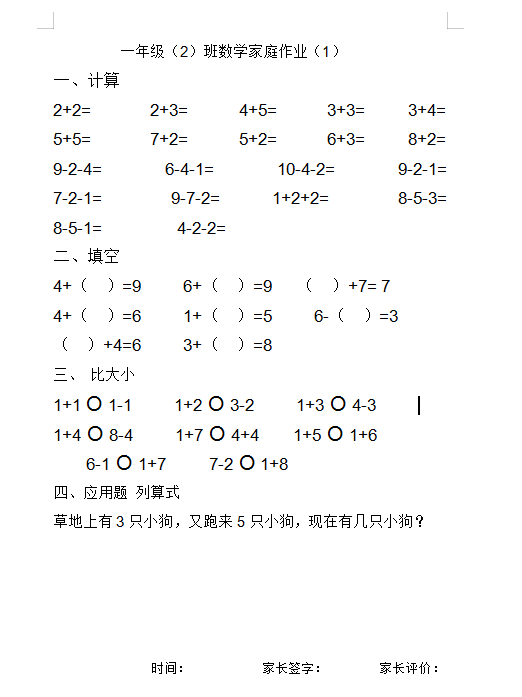2+2=       2+3=      4+5=      3+3=     3+4=

5+5=       7+2=      5+2=      6+3=     8+2=

9-2-4=     6-4-1=     10-4-2=     9-2-1=     7-2-1=    9-7-2=   1+2+2=    8-5-3=      8-5-1=         4-2-2=